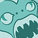# QlikView App Dev

Discussion Board for collaboration related to QlikView App Development.

Announcements
Join us at the Cloud Data and Analytics Tour! REGISTER TODAY
cancel
Showing results for
Search instead for
Did you mean:Contributor II

## Bar Chart Rolling Month Set Analysis don't work

Hi at all,

i have a issue or understanding problem in my bar chart. I have this following formel with expression:

Sum({<%_Key_Account={"ADDEDVALUE"},%_Key_Category={"ACTUAL"},DataMeasures={"PER"},YearMonth ={">=\$(=Date(addmonths(Max(YearMonth), -18), 'MMM-YY')) <=\$(=Date(addmonths(Max(YearMonth), -1), 'MMM-YY'))"}>} DataValue)

For testing i created listboxes with the

%_Key_Account

%_Key_Category

DataMeasures

i selected the values like in the formel above. Everything worked fine, but when i deselect one of this listboxes which i want to filter in my formel, then the set analysis didn't work.

Can anyone help me?

Thanks

1 Solution

Accepted SolutionsContributor II
Author

I found the solution which will work:

Sum({< %_Key_Account={"ADDEDVALUE"},%_Key_Category={"ACTUAL"},DataMeasures={"PER"},YearMonth ={">=\$(=Date(addmonths(Max({<%_Key_Account={ADDEDVALUE},%_Key_Category={ACTUAL},DataMeasures={PER}>}YearMonth), -18), 'MMM-YY')) <=\$(=Date(addmonths(Max({<%_Key_Account={ADDEDVALUE},%_Key_Category={ACTUAL},DataMeasures={PER}>}YearMonth), -1), 'MMM-YY'))"}>} DataValue)/1000000

I must add the filter into the rolling filter

1 ReplyContributor II
Author

I found the solution which will work:

Sum({< %_Key_Account={"ADDEDVALUE"},%_Key_Category={"ACTUAL"},DataMeasures={"PER"},YearMonth ={">=\$(=Date(addmonths(Max({<%_Key_Account={ADDEDVALUE},%_Key_Category={ACTUAL},DataMeasures={PER}>}YearMonth), -18), 'MMM-YY')) <=\$(=Date(addmonths(Max({<%_Key_Account={ADDEDVALUE},%_Key_Category={ACTUAL},DataMeasures={PER}>}YearMonth), -1), 'MMM-YY'))"}>} DataValue)/1000000

I must add the filter into the rolling filter